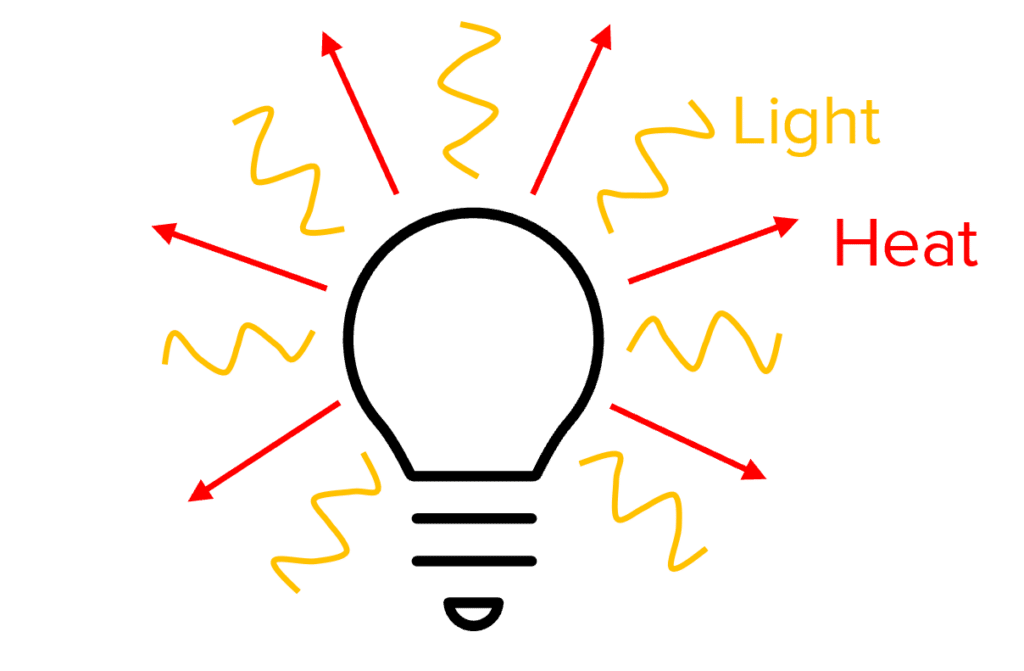# Efficiency

GCSEAQACombined Science FoundationCombined Science HigherPhysics FoundationPhysics Higher

## Efficiency

Efficiency tells us how much energy is transferred to useful energy and how much energy is wasted. By reducing wasted energy, the efficiency of an energy transfer can be increased. This will save energy and money.

## Efficiency Calculations

If you know the amount of input energy and useful energy from an energy transfer, you can calculate the efficiency using the following equation:

$\color{aa57ff}{\text{efficiency}}$$\color{aa57ff}{=}$$\color{aa57ff}{\dfrac{\text{useful output energy}}{\text{total input energy}}}$

Remember that the total energy is equal to the sum of the useful energy and the wasted energy.

$\color{aa57ff}{\text{total energy}}$$\color{aa57ff}{=}$$\color{aa57ff}{\text{useful energy} + }$$\color{aa57ff}{\text{wasted energy}}$

You can also calculate efficiency using the total power input and the useful power input using the following equation:

$\color{aa57ff}{\text{efficiency}}$$\color{aa57ff}{=}$$\color{aa57ff}{\dfrac{\text{useful power output}}{\text{total power input}}}$

GCSECombined Science FoundationCombined Science HigherPhysics FoundationPhysics HigherAQA

## Increasing Efficiency

The efficiency of an energy transfer can be increased by reducing the amount of wasted energy. How we do this depends on the system and the types of energy store involved.

For example, by using oil as a lubricant in a car engine, less heat is produced by friction. Therefore, less energy is wasted as heat and so more energy is converted to useful energy and the engine is more efficient

We always want to make a process more efficient because this will save energy and money

GCSECombined Science HigherPhysics HigherAQA

## Example: Calculating EfficiencyA lightbulb is switched on for a period of time. During this time, the lightbulb uses $100\text{ J}$ of energy and $20\text{ J}$ of heat energy is produced by the lightbulb.

Calculate the efficiency of the lightbulb.

[3 marks]

$\text{useful energy output} = \text{total input energy} - \text{wasted energy output} \\= \textcolor{f21cc2}{100}-\textcolor{00d865}{20} = \bold{80\text{ J}}\\ \text{efficiency}= \dfrac{\text{useful energy output}}{\text{total energy input}}=\dfrac{80\text{ J}}{\textcolor{f21cc2}{100\text{ J}}}= \bold{80\%}\,(\text{or }0.8)$
GCSECombined Science FoundationCombined Science HigherPhysics FoundationPhysics HigherAQA

## Efficiency Example Questions

$\bold{\text{efficiency}} = \dfrac{\text{useful output power}}{\text{total input power}}=\dfrac{4}{6}=\bold{67\%}\text{(2 sf) or } 0.67$Gold Standard Education

$\text{useful energy output} = \text{total energy input} - \text{waste energy output} = 100\%-30\% = \bold{70\%}$Gold Standard Education

Any one from:

• Increase the thickness of the walls of the kettle
• Make the kettle from a more insulating material
• Wrap the kettle in an insulating material (cotton wool, bubble wrap etc).Gold Standard Education

## Efficiency Worksheet and Example Questions

### Energy Changes in a System Questions

GCSEOfficial MMEGold Standard Education

£19.99 /month. Cancel anytime